Tamil Nadu Board of Secondary EducationSSLC (English Medium) Class 7th
Advertisement Remove all ads

# Samacheer Kalvi solutions for Mathematics Term 2 - Class 7th Tamil Nadu State Board chapter 5 - Information Processing [Latest edition]

Advertisement Remove all ads

#### Chapters## Chapter 5: Information Processing

Exercise 5.1Exercise 5.2Exercise 5.3
Exercise 5.1 [Pages 92 - 93]

### Samacheer Kalvi solutions for Mathematics Term 2 - Class 7th Tamil Nadu State Board Chapter 5 Information Processing Exercise 5.1 [Pages 92 - 93]

Exercise 5.1 | Q 1 | Page 92

Match the given patterns of shapes with the appropriate number pattern and its generalization.

 Column A Column B (i)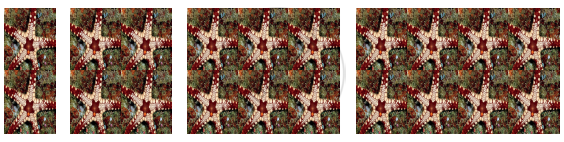(a) Sequence: 5, 9, 13, 17 ... General form: y = 4n + 1 (ii)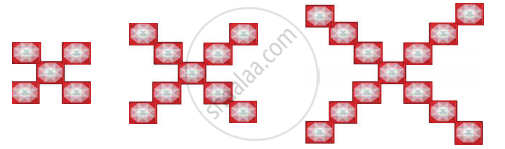(b) Sequence: 3, 4, 5, 6, ... General form: y = x + 2 (iii)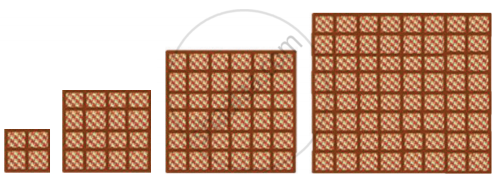(c) Sequence: 1, 4, 9, 16 ... General form: y = n2 (iv)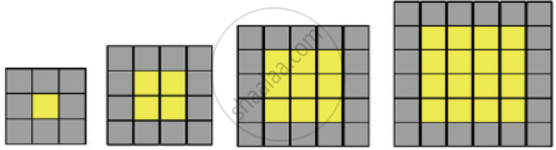(d) Sequence: 2, 4, 6, 8 ... General form: y = 2n (v)(e) Sequence: 4, 16, 36, 64 ... General form: y = 4n2

#### Objective type questions

Exercise 5.1 | Q 2 | Page 93

Identify the correct relationship between x andy from the given table.

 x 1 2 3 4 ... y 4 8 12 16 ...
• y = 4x

• y = x + 4

• y = 4

• y = 4 × 4

Exercise 5.1 | Q 3 | Page 93

Identify the correct relationship between x and y from the given table.

 x −2 −1 0 1 2 ... y 6 3 0 −3 −6 ...
• y = −2x

• y = +2x

• y = +3x

• y = −3x

Advertisement Remove all ads
Exercise 5.2 [Page 97]

### Samacheer Kalvi solutions for Mathematics Term 2 - Class 7th Tamil Nadu State Board Chapter 5 Information Processing Exercise 5.2 [Page 97]

Exercise 5.2 | Q 1 | Page 97

Complete the Pascal’s Triangle.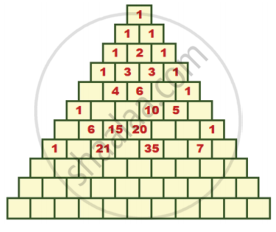Exercise 5.2 | Q 2. (i) | Page 97

The following hexagonal shapes are taken from Pascal’s Triangle. Fill in the missing numbers.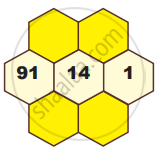Exercise 5.2 | Q 2. (ii) | Page 97

The following hexagonal shapes are taken from Pascal’s Triangle. Fill in the missing numbers.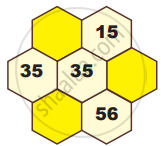Exercise 5.2 | Q 2. (iii) | Page 97

The following hexagonal shapes are taken from Pascal’s Triangle. Fill in the missing numbers.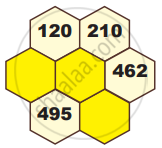Exercise 5.2 | Q 2. (iv) | Page 97

The following hexagonal shapes are taken from Pascal’s Triangle. Fill in the missing numbers.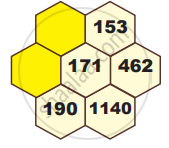Exercise 5.2 | Q 3 | Page 97

Complete the Pascal’s Triangle by taking the numbers 1, 2, 6, 20 as line of symmetry.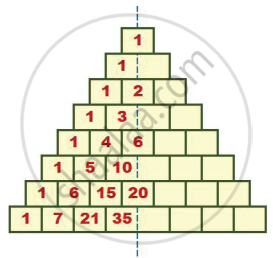#### Objective type questions

Exercise 5.2 | Q 4 | Page 97

The elements along the sixth row of the Pascal’s Triangle is

• 1, 5, 10, 5, 1

• 1, 5, 5, 1

• 1, 5, 5, 10, 5, 5, 1

• 1, 5, 10, 10, 5, 1

Exercise 5.2 | Q 5 | Page 97

The difference between the consecutive terms of the fifth slanting row containing four elements of a Pascal’s Triangle is

• 3, 6, 10, …

• 4, 10, 20, …

• 1, 4, 10, …

• 1, 3, 6, …

Exercise 5.2 | Q 6 | Page 97

What is the sum of the elements of nineth row in the Pascal’s Triangle?

• 128

• 254

• 256

• 126

Advertisement Remove all ads
Exercise 5.3 [Page 98]

### Samacheer Kalvi solutions for Mathematics Term 2 - Class 7th Tamil Nadu State Board Chapter 5 Information Processing Exercise 5.3 [Page 98]

#### Miscellaneous Practice problems

Exercise 5.3 | Q 1 | Page 98

Choose the correct relationship between x and y from the given table.

 x −2 −1 0 1 2 ... y 4 5 6 7 8 ...
• y = x + 4

• y = x + 5

• y = x + 6

• y = x + 7

Exercise 5.3 | Q 2 | Page 98

Find the triangular numbers from the Pascal’s Triangle and colour them.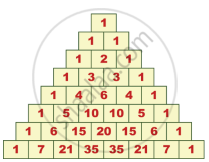Exercise 5.3 | Q 3 | Page 98

Write the first five numbers in the third slanting row of the Pascal’s Triangle and find their squares. What do you infer?

#### Challenge Problems

Exercise 5.3 | Q 4. (i) | Page 98

Tabulate and find the relationship between the variables (x and y) for the following patterns.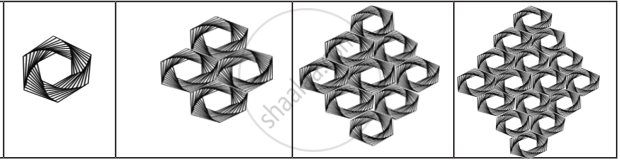Exercise 5.3 | Q 4. (ii) | Page 98

Tabulate and find the relationship between the variables (x and y) for the following patterns.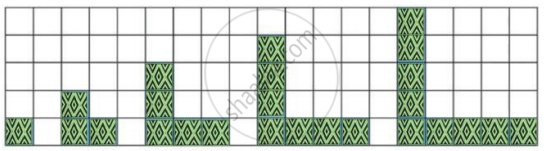Exercise 5.3 | Q 5. (i) | Page 98

Verify whether the following hexagonal shapes form a part of the Pascal’s Triangle.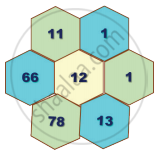Exercise 5.3 | Q 5. (ii) | Page 98

Verify whether the following hexagonal shapes form a part of the Pascal’s Triangle.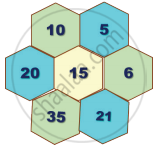Exercise 5.3 | Q 5. (iii) | Page 98

Verify whether the following hexagonal shapes form a part of the Pascal’s Triangle.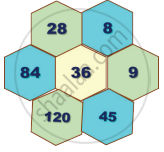Exercise 5.3 | Q 5. (iv) | Page 98

Verify whether the following hexagonal shapes form a part of the Pascal’s Triangle.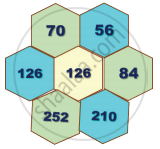Advertisement Remove all ads

## Chapter 5: Information Processing

Exercise 5.1Exercise 5.2Exercise 5.3## Samacheer Kalvi solutions for Mathematics Term 2 - Class 7th Tamil Nadu State Board chapter 5 - Information Processing

Samacheer Kalvi solutions for Mathematics Term 2 - Class 7th Tamil Nadu State Board chapter 5 (Information Processing) include all questions with solution and detail explanation. This will clear students doubts about any question and improve application skills while preparing for board exams. The detailed, step-by-step solutions will help you understand the concepts better and clear your confusions, if any. Shaalaa.com has the Tamil Nadu Board of Secondary Education Mathematics Term 2 - Class 7th Tamil Nadu State Board solutions in a manner that help students grasp basic concepts better and faster.

Further, we at Shaalaa.com provide such solutions so that students can prepare for written exams. Samacheer Kalvi textbook solutions can be a core help for self-study and acts as a perfect self-help guidance for students.

Concepts covered in Mathematics Term 2 - Class 7th Tamil Nadu State Board chapter 5 Information Processing are Tables and Patterns Leading to Linear Functions, Pascal’s Triangle.

Using Samacheer Kalvi Class 7th solutions Information Processing exercise by students are an easy way to prepare for the exams, as they involve solutions arranged chapter-wise also page wise. The questions involved in Samacheer Kalvi Solutions are important questions that can be asked in the final exam. Maximum students of Tamil Nadu Board of Secondary Education Class 7th prefer Samacheer Kalvi Textbook Solutions to score more in exam.

Get the free view of chapter 5 Information Processing Class 7th extra questions for Mathematics Term 2 - Class 7th Tamil Nadu State Board and can use Shaalaa.com to keep it handy for your exam preparation

Advertisement Remove all ads
Share
Notifications

View all notifications

Forgot password?